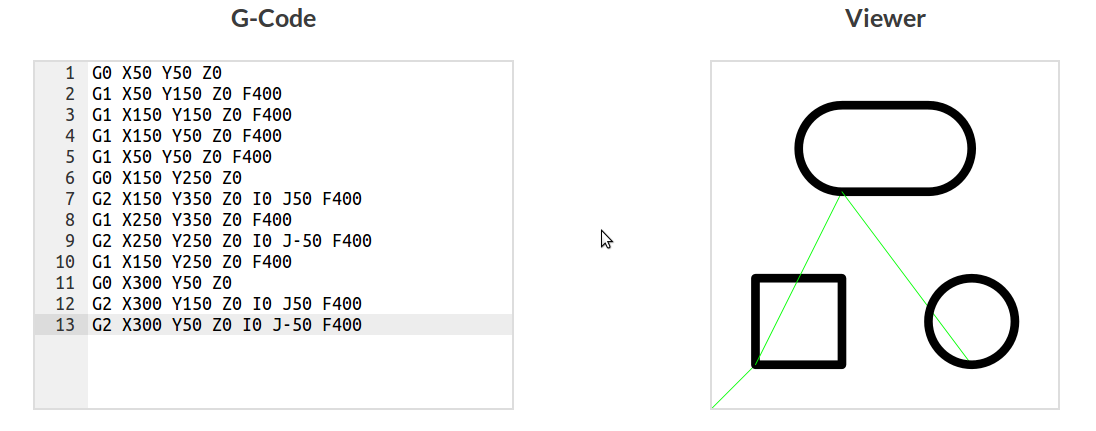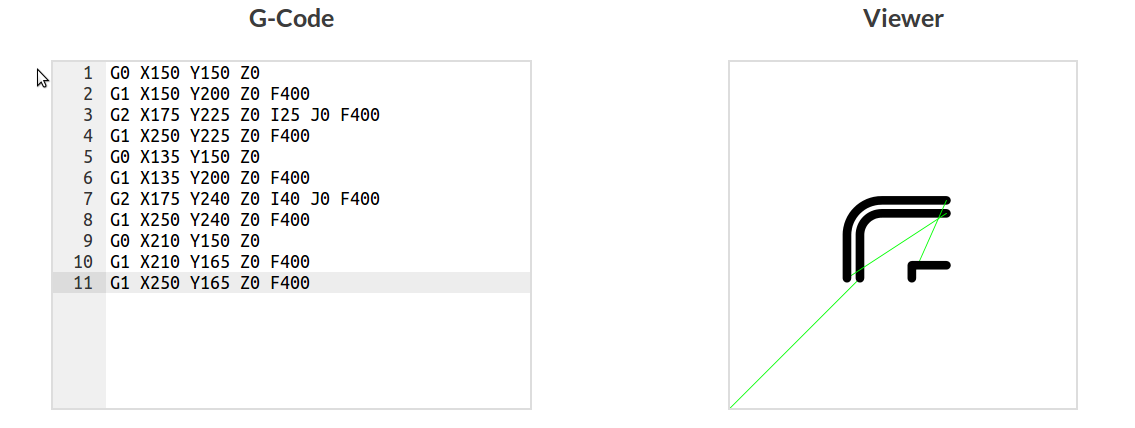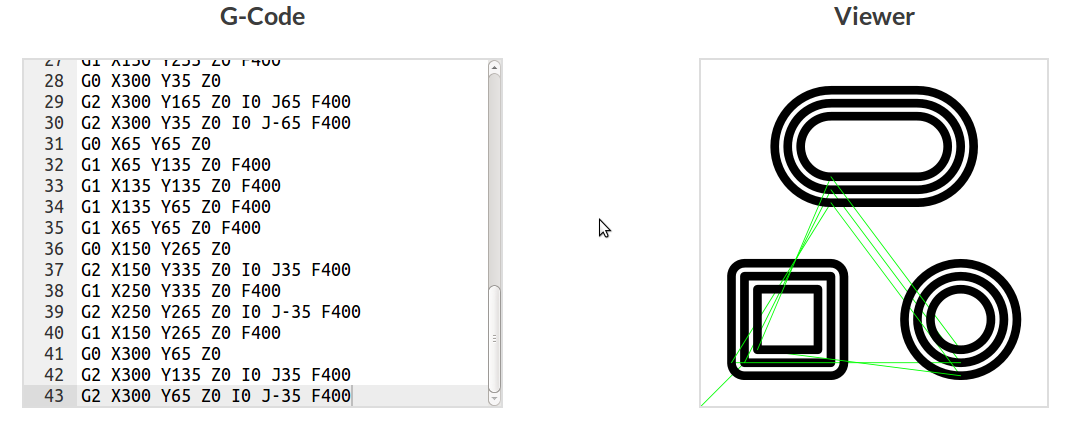Published: 10-06-2016
Programatically milling profiles. Looking at: Geometry generation of profiles, which consist of arcs and lines. An offsetting algorithm for transforming the profiles. Generating G-code and rendering it to SVG.
Contents
Overview
The aim is to write some code to aid creating profiles. In this context a profile is a collection of segments. Each segment can be a line or an arc. There also needs to be a way of offsetting the profile, both inside and and out. This is to allow for cutter diameter when design. As it turns out the offsetting is the hardest part.
Data primatives
The basic data primitives are the point, line and arc. These might be better explained with some typescript:

interface Point {
x: number,
y: number,
z?: number
}

interface Line {
type: 'line',
start: Point,
end: Point
}

interface Arc {
type: 'arc',
clockwise: boolean,
start: Point,
end: Point,
center: Point
}


All the details of the profile will be stored in a object. Here typescript classes are used to create and manage the objects, but really it's exactly the same as creating a new object and attaching methods to the prototype. A new profile object can be initiated with it's starting point and then lines and arcs can be appended.
Method chaining
Using method chaining allows the user to write very readable code. The chaining is created by returning 'this' from the method. The idea is to be able to create a profile using the style shown below:

// New profile with start points x,y
var profile = new Profile(x, y);
// Setting segments using method chaining
profile.line(x, y).arc(x, y, direction, cx, cy).line(x, y);
// Get all segments
profile.dump();


When entering details about the line or arc segment, only the end point is needed, the start point can be calculated from the previous line/arc/start point. This makes it easier for the user. Start and end points are needed on each segment for some of the processing done later with offsets. The basic profile class looks like this:

export class Profile {

segments: (Line | Arc)[] = [];
last_end: Point;
constructor(x: number, y: number) {
this.last_end = { x: x, y: y };
}

line(x: number, y: number) {
this.segments.push({
type: 'line',
start: { x: this.last_end.x, y: this.last_end.y },
end: { x: x, y: y }
});
this.last_end = { x: x, y: y };
return this;
}

arc(x: number, y: number, clockwise: boolean, cx: number, cy: number) {
var absolute_center: Point = { x: this.last_end.x + cx, y: this.last_end.y + cy };
this.segments.push({
type: 'arc',
clockwise: clockwise,
start: { x: this.last_end.x, y: this.last_end.y },
end: { x: x, y: y },
center: absolute_center
});
this.last_end = { x: x, y: y };
return this;
}

dump() {
return this.segments;
}
}


The last_end global variable keeps track of the previous end point for so the start point can be added to the next line/arc.
Rendering the profile
Instead of rendering the raw profile, it will be converted straight to G-code and plotted using the G-code viewer built previously. So, really we are performing a very basic CAM profile operation. The following class was written to convert the geometric objects to G-code.

export class Geom2Gcode {

commands: string[];

constructor() {
this.commands = [];
}

moveG(segment: any) {
return G0 X${segment.start.x} Y${segment.start.y} Z0;
}

lineG(line: any) {
return G1 X${line.end.x} Y${line.end.y} Z0 F400;
}

arcG(arc: any) {
var direction = arc.clockwise ? 'G2' : 'G3'
return ${direction} X${arc.end.x} Y${arc.end.y} Z0 I${arc.center.x - arc.start.x} J\${arc.center.y - arc.start.y} F400;
}

profile(segments: (Line | Arc)[]) {
this.commands.push(this.moveG(segments));
this.commands = this.commands.concat(segments.map((segment) => {
switch (segment.type) {
case 'line':
return this.lineG(segment);
case 'arc':
return this.arcG(segment);
}
}));
return this;
}

gcode() {
return this.commands;
}
}


Drawing something
Lets draw some basic shapes using the Profile class.

var square_profile = new Profile(50, 50);
square_profile
.line(50, 150)
.line(150, 150)
.line(150, 50)
.line(50, 50);

var arc_profile = new Profile(150, 250);
arc_profile
.arc(150, 350, true, 0, 50)
.line(250, 350)
.arc(250, 250, true, 0, -50)
.line(150, 250);

var circle_profile = new Profile(300, 50);
circle_profile
.arc(300, 150, true, 0, 50)
.arc(300, 50, true, 0, -50);

var gcode = new Geom2Gcode();
gcode.profile(square_profile.dump());
gcode.profile(arc_profile.dump());
gcode.profile(circle_profile.dump());

console.log(gcode.gcode().join('\n'));

// G0 X50 Y50 Z0
// G1 X50 Y150 Z0 F400
// G1 X150 Y150 Z0 F400
// G1 X150 Y50 Z0 F400
// G1 X50 Y50 Z0 F400
// G0 X150 Y250 Z0
// G2 X150 Y350 Z0 I0 J50 F400
// G1 X250 Y350 Z0 F400
// G2 X250 Y250 Z0 I0 J-50 F400
// G1 X150 Y250 Z0 F400
// G0 X300 Y50 Z0
// G2 X300 Y150 Z0 I0 J50 F400
// G2 X300 Y50 Z0 I0 J-50 F400Drawing basic profiles.
Offset algorithm
• Shift all lines and arcs by offset, delete arcs if radius becomes <= 0
• Check for intersections/gaps
• If gap fill with arc
• If intersection, trim lines
Shifting lines
The first step in the offset algorithm is to shift all the lines/arcs. To shift a line, first you need its unit vector. From this its normal unit vector can be found by rotating through 90 degrees anti-clockwise. To shift the line we simply add the offset multiplied by the normal unit vector to both the start and end points. When the offset is positive the line is shifted outwards, and when negative shifted inwards.
Shifting arcs
A same approach used for shifting lines is used for arcs. Unit vectors are calculated for the center to start points and the center to end point. Then vectors are multiplied by the offsets and added to the start and end points. The center location is kept the same. To aid in maintaining the same center point absolute coordinates are used to represent arcs internally. The relative coordinates are calculated again during the G-code conversion.
Finding and checking intersections
When shifting lines inwards (negative offset) they will begin to overlap. To get rid of this overlap the intersection point needs to be located.
Initially I tried to use the standard equation of a line y = mx + c but realised that was a bad idea. When using the equation in this form there are a few common edge cases which don't work well computationally. It requires lots of 'if' statements to correctly handle gradients of 0 and infinity. Instead the general equation of a line Ax + BY = C proved more useful. The coefficients are calculated using:
$$A = y_2 - y_1$$
$$B = x_1 - x_2$$
$$C = Ax_1 + By_1$$
The intersections can be found by solving for x and y. This can done using the dot product. Here we use it explicitly in equation form rather than using vectors.

checkIntersect(current: (Line | Arc), next: (Line | Arc)): Intersection {
var x: number;
var y: number;
var A1 = current.end.y - current.start.y;
var B1 = current.start.x - current.end.x;
var C1 = (A1 * current.start.x) + (B1 * current.start.y);
var A2 = next.end.y - next.start.y;
var B2 = next.start.x - next.end.x;
var C2 = (A2 * next.start.x) + (B2 * next.start.y);

var det = (A1 * B2) - (A2 * B1);
// Check for parallel lines
if (det === 0) {
return {
true: false,
location: { x: 0, y: 0 }
}
} else {
x = ((B2 * C1) - (B1 * C2)) / det // Find x intersection point
y = ((A1 * C2) - (A2 * C1)) / det // Find y intersection point

var x_check1: boolean = Math.min(current.start.x, current.end.x) <= x && x <= Math.max(current.start.x, current.end.x);
var y_check1: boolean = Math.min(current.start.y, current.end.y) <= y && y <= Math.max(current.start.y, current.end.y);
var x_check2: boolean = Math.min(next.start.x, next.end.x) <= x && x <= Math.max(next.start.x, next.end.x);
var y_check2: boolean = Math.min(next.start.y, next.end.y) <= y && y <= Math.max(next.start.y, next.end.y);

// If intersects
if (x_check1 && y_check1 && x_check2 && y_check2) {
return {
true: true,
location: { x: x, y: y }
}
} else {
return {
true: false,
location: { x: x, y: y }
}
}

}
}


Trimming intersections
If the two lines are found to intersect the end of the first line and start of the second line are set to the intersection coordinates. This effectively 'trims' off any excess line length.Trimming intersections
Joining gaps
When lines are moved outwards (positive offset) there will be a gap between the end and start points. To maintain the correct geometry these points need to be joined used an arc. If the line intersection checker returns false a new arc is created with the corresponding start and end points of its neighbouring lines. The arc's center point is set to the origin intersection point of the two lines before the offset.Joining gaps
Arc radius < 0
When an arc is offset inwards far enough the radius might become negative. In this case we want to change the arc to a point to prevent weird geometry. To do this we check whether the components of the unit vector have changed after the shift. If they have, the line has changed direction and the arc is not added to the shifted array. An example of a correctly 'destroyed' arc is shown below.Result of an arc radius reduced below 0.
Drawing with offsets
Drawing the earlier profile example with both positive and negative offsets is done simply by calling the shift() method as shown in the code below:

var square_profile = new Profile(50, 50);
square_profile
.line(50, 150)
.line(150, 150)
.line(150, 50)
.line(50, 50);

var arc_profile = new Profile(150, 250);
arc_profile
.arc(150, 350, true, 0, 50)
.line(250, 350)
.arc(250, 250, true, 0, -50)
.line(150, 250);

var circle_profile = new Profile(300, 50);
circle_profile
.arc(300, 150, true, 0, 50)
.arc(300, 50, true, 0, -50);

var gcode = new Geom2Gcode();
gcode.profile(square_profile.dump());
gcode.profile(arc_profile.dump());
gcode.profile(circle_profile.dump());

gcode.profile(square_profile.shift(15));
gcode.profile(arc_profile.shift(15));
gcode.profile(circle_profile.shift(15));

gcode.profile(square_profile.shift(-15));
gcode.profile(arc_profile.shift(-15));
gcode.profile(circle_profile.shift(-15));

console.log(gcode.gcode().join('\n'));Adding offsets to the basic profile.
The full code

interface Point {
x: number,
y: number,
z?: number
}

interface Line {
type: 'line',
start: Point,
end: Point,
unit: [number, number]
}

interface Arc {
type: 'arc',
clockwise: boolean,
start: Point,
end: Point,
center: Point,
start_unit: [number, number],
end_unit: [number, number]
}

interface Intersection {
true: boolean,
location: Point
}

export class Profile {
/**
*	@param x This is a Profile class
*
**/
segments: (Line | Arc)[] = [];
last_end: Point;
constructor(x: number, y: number) {
this.last_end = { x: x, y: y };
}

line(x: number, y: number) {
var dy: number = (y - this.last_end.y);
var dx: number = (x - this.last_end.x);
var length = Math.sqrt((dy * dy) + (dx * dx));
var unit: [number, number] = [dx / length, dy / length];
this.segments.push({
type: 'line',
start: { x: this.last_end.x, y: this.last_end.y },
end: { x: x, y: y },
unit: unit
});
this.last_end = { x: x, y: y };
return this;
}

arc(x: number, y: number, clockwise: boolean, cx: number, cy: number) {
var absolute_center: Point = { x: this.last_end.x + cx, y: this.last_end.y + cy };
var start_dx = this.last_end.x - absolute_center.x;
var start_dy = this.last_end.y - absolute_center.y;
var length = Math.sqrt((start_dy * start_dy) + (start_dx * start_dx));
var end_dx = x - absolute_center.x;
var end_dy = y - absolute_center.y;
var start_unit: [number, number] = [start_dx / length, start_dy / length];
var end_unit: [number, number] = [end_dx / length, end_dy / length];
this.segments.push({
type: 'arc',
clockwise: clockwise,
start: { x: this.last_end.x, y: this.last_end.y },
end: { x: x, y: y },
center: absolute_center,
start_unit: start_unit,
end_unit: end_unit
});
this.last_end = { x: x, y: y };
return this;
}

rotateUnitVector90(unit) {
var new_x: number = -unit;
var new_y: number = unit;
return [new_x, new_y];
}

shift(offset: number) {
/*
Algorithm:
Map through and move lines and arcs along unit normals by offset
- If arc radius < 0 remove
Map through again finding intersections or gaps
- If intersection, trim
- If gap, join
return new geometry array
*/

// Shift start and end points
var movedPoints: (Line | Arc)[] = [];
this.segments.forEach((segment: (Line | Arc), index) => {

switch (segment.type) {

case ('line'):
var rtu = this.rotateUnitVector90(segment.unit); // Rotate unit vector
var new_end_x: number = segment.end.x + rtu * offset;
var new_end_y: number = segment.end.y + rtu * offset;
var new_start_x: number = segment.start.x + rtu * offset;
var new_start_y: number = segment.start.y + rtu * offset;
movedPoints.push({
type: 'line',
start: { x: new_start_x, y: new_start_y },
end: { x: new_end_x, y: new_end_y },
unit: segment.unit
});
break;
case ('arc'):
var absolute_center = segment.center;
var previous_line = this.segments[index - 1];
var new_start_x: number = segment.start.x + segment.start_unit * offset;
var new_start_y: number = segment.start.y + segment.start_unit * offset;
var new_end_x: number = segment.end.x + segment.end_unit * offset;
var new_end_y: number = segment.end.y + segment.end_unit * offset;

movedPoints.push({
type: 'arc',
clockwise: segment.clockwise,
start: { x: new_start_x, y: new_start_y },
end: { x: new_end_x, y: new_end_y },
center: { x: absolute_center.x, y: absolute_center.y },
start_unit: segment.start_unit,
end_unit: segment.end_unit
});
break;
}
});

var cleaned: (Line | Arc)[] = [];
// Check if profile is closed or open
var closed: boolean = (this.segments.start.x === this.segments.slice(-1).end.x && this.segments.start.y === this.segments.slice(-1).end.y);

movedPoints.forEach((segment, index) => {
var last: boolean = (index === movedPoints.length - 1);
var original: (Line | Arc);
var current: (Line | Arc);
var next: (Line | Arc);

original = this.segments[index];
current = movedPoints[index];
next = movedPoints[index + 1];
if (closed && last) {
original = this.segments[index];
current = movedPoints[index];
next = movedPoints;
} else if (!closed && last) {
cleaned.push(current);
return
}

switch (segment.type) {
case 'line':
if (next.type === 'arc') {
cleaned.push(current);
return;
}
var intersect: Intersection = this.checkIntersect(current, next);
if (intersect.true) {
current.end.x = intersect.location.x;
current.end.y = intersect.location.y;
next.start.x = intersect.location.x;
next.start.y = intersect.location.y;
cleaned.push(current);
} else {
cleaned.push(segment);
var clockwise = offset > 0 ? true : false;
var new_arc: Arc = {
type: 'arc',
clockwise: clockwise,
start: current.end,
end: next.start,
center: this.segments[index].end,
start_unit: current.start_unit,
end_unit: current.end_unit
};
cleaned.push(new_arc);
}
break;
case 'arc':
cleaned.push(current);
break;
}
});
return cleaned;
}

checkIntersect(current: (Line | Arc), next: (Line | Arc)): Intersection {
var x: number;
var y: number;
var A1 = current.end.y - current.start.y;
var B1 = current.start.x - current.end.x;
var C1 = (A1 * current.start.x) + (B1 * current.start.y);
var A2 = next.end.y - next.start.y;
var B2 = next.start.x - next.end.x;
var C2 = (A2 * next.start.x) + (B2 * next.start.y);

var det = (A1 * B2) - (A2 * B1);
// Check for parallel lines
if (det === 0) {
return {
true: false,
location: { x: 0, y: 0 }
}
} else {
x = ((B2 * C1) - (B1 * C2)) / det // Find x intersection point
y = ((A1 * C2) - (A2 * C1)) / det // Find y intersection point

var x_check1: boolean = Math.min(current.start.x, current.end.x) <= x && x <= Math.max(current.start.x, current.end.x);
var y_check1: boolean = Math.min(current.start.y, current.end.y) <= y && y <= Math.max(current.start.y, current.end.y);
var x_check2: boolean = Math.min(next.start.x, next.end.x) <= x && x <= Math.max(next.start.x, next.end.x);
var y_check2: boolean = Math.min(next.start.y, next.end.y) <= y && y <= Math.max(next.start.y, next.end.y);

// If intersects
if (x_check1 && y_check1 && x_check2 && y_check2) {
return {
true: true,
location: { x: x, y: y }
}
} else {
return {
true: false,
location: { x: x, y: y }
}
}

}
}

lines(points: any) {
points.forEach((line) => {
this.line(line.x, line.y);
});
return this;
}

dump() {
return this.segments;
}
}